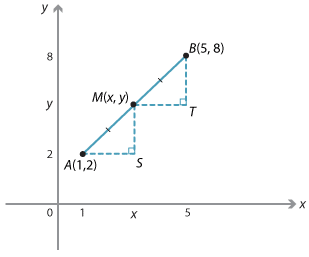When the interval is not parallel to one of the axes we take the average of the x-coordinates and the y-coordinates.Detailed description

#### Example 2

Let M be the midpoint of the line AB. Triangles AMS and MBT are congruent triangles (AAS), and so $$AS = MT$$ and $$MS = BT$$.

Hence the x-coordinate of M is the average of 1 and 5.

$$x = \dfrac{5 + 1}{2} = 3$$

The y-coordinate of M is the average of 2 and 8.

$$x = \dfrac{2 + 8}{2} = 5$$

Thus the coordinates of the midpoint M are (3, 5).